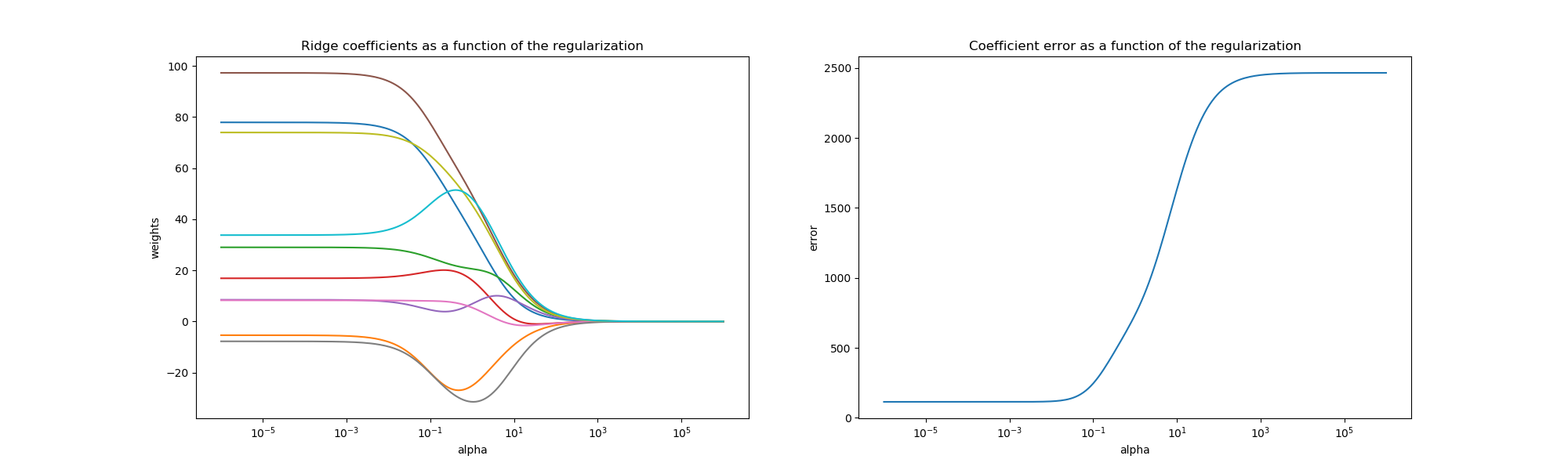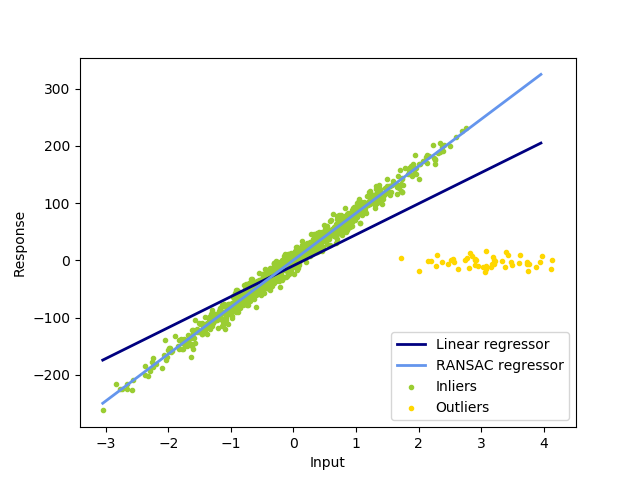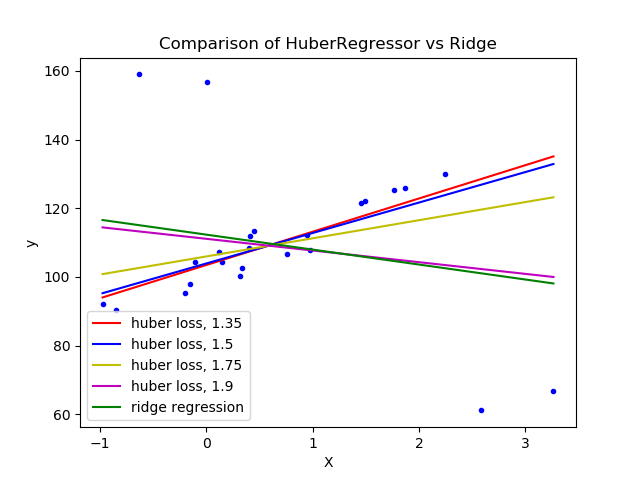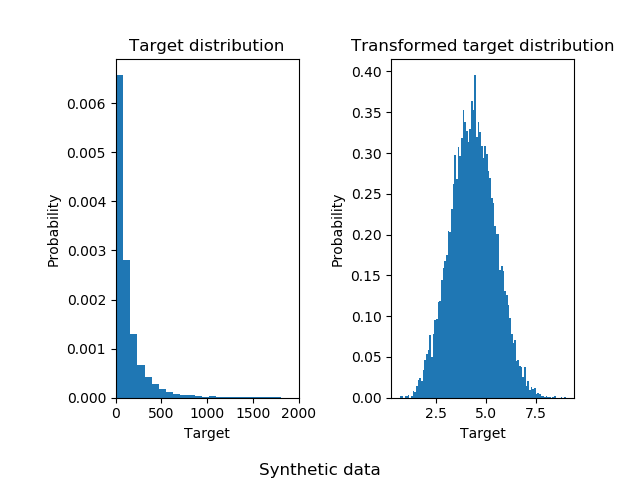# sklearn.datasets.make_regression¶

sklearn.datasets.make_regression(n_samples=100, n_features=100, *, n_informative=10, n_targets=1, bias=0.0, effective_rank=None, tail_strength=0.5, noise=0.0, shuffle=True, coef=False, random_state=None)

[源码]

n_samples int, optional (default=100)

n_features int, optional (default=100)

n_informative int, optional (default=10)

n_targets int, optional (default=1)

bias float, optional (default=0.0)

effective_rank int or None, optional (default=None)
- 如果不是None:

- if None:

tail_strength float between 0.0 and 1.0, optional (default=0.5)

noise float, optional (default=0.0)

shuffle boolean, optional (default=True)
shuffle样本和特征。
coef boolean, optional (default=False)

random_state int, RandomState instance, default=None

X array of shape [n_samples, n_features]

y array of shape [n_samples] or [n_samples, n_targets]

coef array of shape [n_features] or [n_features, n_targets], optional

## sklearn.datasets.make_regression使用示例¶